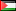##### On the Numerical Treatment of Heat Conduction Problem by Boundary Element and Multigrid Methods
Publication Type
Original research
Authors

In this work we consider the boundary integral equation describing the

steady state heat conduction taking place in three dimensional enclosure

geometries. For the numerical realization of the Fredholm integral equation,

we use the boundary element method based on the Galerkin weighted

residuals method. Consequently, converting the original integral equation

into a set of algebraic equations. We apply the multigrid iterations to solve

the system of linear equations. To demonstrate the efficiency of this iterative

scheme, we construct numerical example.

Keywords: Heat conduction, Fredholm integral equation, Boundary

element method, Multigrid iterations.

.

Journal
Title
An-Najah Univ. J. Res. (N. Sc)
Publisher
An-Najah National University
Publisher Country
PalestinePublication Type
Both (Printed and Online)
Volume
33
Year
2019
Pages
--